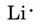# Lewis Diagrams and Biological and Chemical Properties

### Lewis Diagrams in Biology

A first step toward gaining some insight into the biological properties of atoms or compounds is to draw their Lewis diagrams. For example, we'll explore lithium fluoride and sodium chloride below. Lithium fluoride and sodium chloride are similar in some ways: The both contain 1+ alkali metal ions and 1- halogen ions; they both are high melting, white, crystalline solids; and they both dissolve in water to make conducting solutions.

But as we have come to suspect, the biological properties are quite different, and must be considered along with chemical properties. Lithium Fluoride is very toxic Because of the lithium ion's small size, it causes ion (electrolyte) imbalances, and, of course, is a strong sedative. Fluoride ion forms hydrofluoric acid in the stomach which is very corrosive, and disrupts metabolism. Sodium chloride (table salt) is much less toxic, with high doses leading to chronic high blood pressure.

G.N. Lewis used simple diagrams (now called "Lewis Diagrams") to keep track of how many electrons were present in the outermost, or valence, shell of a given atom. The kernel of the atom, i.e., the nucleus together with the inner electrons, is represented by the chemical symbol, and only the valence electrons are drawn as dots surrounding the chemical symbol. Thus the three atoms shown here from Fig. 1 from Electrons and Valence can be represented by the following Lewis diagrams:Figure $$\PageIndex{1}$$ The shell structure of atoms of He, Cl, and K, as suggested by Lewis.If the atom is a noble-gas atom, two alternative procedures are possible. Either we can consider the atom to have zero valence electrons or we can regard the outermost filled shell as the valence shell. The first three noble gases can thus be written asExample $$\PageIndex{1}$$: Lewis Diagrams

Draw Lewis diagrams for an atom of each of the following elements:

Li N F Na

Solution We find from the periodic table inside the front cover that Li has an atomic number of 3. It thus contains three electrons, one more than the noble gas He. This means that the outermost, or valence, shell contains only one electron, and the Lewis diagram isFollowing the same reasoning, N has seven electrons, five more than He, while F has nine electrons, seven more than He, givingNa has nine more electrons than He, but eight of them are in the kernel, corresponding to the eight electrons in the outermost shell of Ne. Since Na has only 1 more electron than Ne, its Lewis diagram isNotice from the preceding example that the Lewis diagrams of the alkali metals are identical except for their chemical symbols. This agrees nicely with the very similar chemical behavior of the alkali metals. Similarly, Lewis diagrams for all elements in other groups, such as the alkaline earths or halogens, look the same. The Lewis diagrams may also be used to predict the valences of the elements. Lewis suggested that the number of valences of an atom was equal to the number of electrons in its valence shell or to the number of electrons which would have to be added to the valence shell to achieve the electronic shell structure of the next noble gas. As an example of this idea, consider the elements Be and O. Their Lewis diagrams and those of the noble gases He and Ne areComparing Be with He, we see that the former has two more electrons and therefore should have a valence of 2. The element O might be expected to have a valence of 6 or a valence of 2 since it has six valence electrons—two less than Ne. Using rules of valence developed in this way, Lewis was able to account for the regular increase and decrease in the subscripts of the compounds in the table below. For example, he reasoned that lithium would lose an electron to fluorine, forming toxic Li+F-:Figure $$\PageIndex{2}$$ Formation of ions by achieving inert gas structures

Or that chlorine would gain an electron from sodium to form Na+Cl-:

http://chemwiki.ucdavis.edu/@api/deki/files/71496/Ionic_bonding_animation.gif

Figure $$\PageIndex{3}$$ Formation of ions by achieving inert gas structures

Similarly, oxygen would gain one electron from each of two sodium ions to form nontoxic Na+2O-, another of the successfully predicted compounds in the table.

In addition he was able to account for more than 50 percent of the formulas in the table. (Those that agree with his ideas are shaded in color or gray in the table. You may wish to refer to that table now and verify that some of the indicated formulas follow Lewis’ rules.) Lewis’ success in this connection gave a clear indication that electrons were the most important factor in holding atoms together when molecules formed.

Table $$\PageIndex{1}$$ Compounds of Hydrogen, Oxygen, and ChlorineDespite these successes, there are also difficulties to be found in Lewis’ theories, in particular for elements beyond calcium in the periodic table. The element Br (Z = 35), for example, has 17 more electrons than the noble-gas Ar (Z = 18). This leads us to conclude that Br has 17 valence electrons, which makes it awkward to explain why Br resembles Cl and F so closely even though these two atoms have only seven valence electrons.

From ChemPRIME: 5.2: Lewis Diagrams